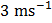# Ifrepresent the work done in moving a particle from A to B along three different paths 1, 2 and 3 respectively(as shown)in the gravitational field of a point mass m. Find the correct relation betweena)b)c)d)## Question ID - 51012 :- Ifrepresent the work done in moving a particle from A to B along three different paths 1, 2 and 3 respectively(as shown)in the gravitational field of a point mass m. Find the correct relation betweena)b)c)d)3537

 (b) Gravitational field is a conservative force field. In a conservative force field work done is path independent.A machine which is 75% efficient uses 12 J of energy in lifting up a 1 kg mass through a certain distance. The mass is then allowed to fall through, that distance. The velocity of the ball at the end of its fall is a)b)c)d)